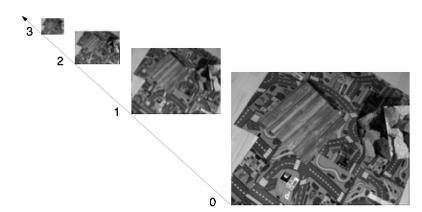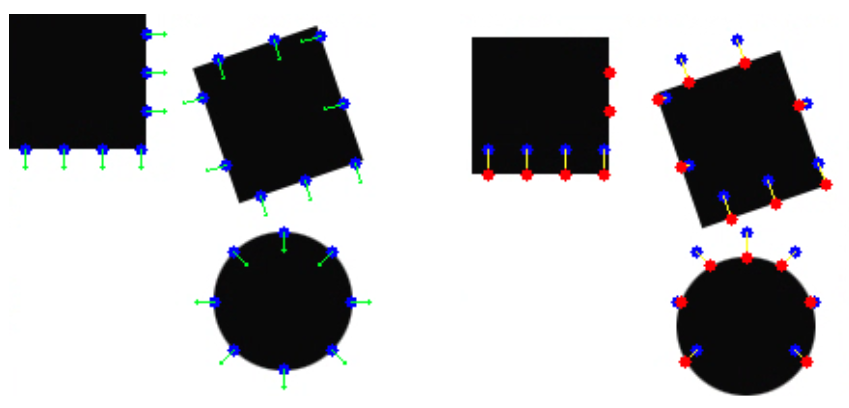##### 计算步骤

(1) 分别对图像$I$和图像$J$建立金字塔${I^L} _{L=0,…,L _m}$和${J^L} _{L=0,…,L _m}$，$L _m$为给定的金字塔层数，一般为3（图像金字塔化一般包括两个步骤：首先对图像进行一次低通滤波进行平滑，然后对图像的横纵两个方向1/2抽样，从而得到一系列尺度缩小的图像。当L＝0时，为原图，当向金字塔的上层移动时，尺寸和分辨率降低，伴随的细节就越少。我们从顶层开始对目标点进行跟踪，先获得一个粗糙的结果，然后将结果作为下一层的初始点再进行跟踪，不断迭代直到到达第0层。这是一种由粗到细分析策略）。(2) 初始化顶层金字塔的搜索偏移位置：$\mathbf{g}^{L _m}=[g _{x}^{L _m}\ g _{y}^{L _m }]^T =[0\ 0]^T$

(3) 从第$L=L _m$层(顶层)金字塔图像开始不断往下，对每一层图像作作以下操作：

(3.1) 计算特征点$\mathbf{x}$在金字塔第$L$层图$I^L$上的位置：$\mathbf{x}^L=[p _x\ p _y]^T=\mathbf{x}/2^L$。

(3.2) 计算搜索起始位置$\mathbf{x} _{init}'$在金字塔第$L$层图$J^L$上的位置：$\mathbf{x}'^L=[p' _x\ p' _y]^T=\mathbf{x} _{init}'/2^L$。

(3.3) 计算最速下降矩阵$\mathbf{S}(u,v)=[I _x (p _x+u,p _y+v)\ I _y (p _x+u,p _y+v)]\mathbf{n}$，$(u,v)⊆W$，$\mathbf{S}$矩阵跟窗口$W$大小一致。其中，$I _x (x,y)$，$I _y (x,y)$为图$I^L$在$(x,y)$位置$X$和$Y$两个方向的梯度。

(3.4) 计算在特征点$\mathbf{x}$在第$L$层的空间梯度值$\textstyle m^L = \sum _{(u,v)⊆W} \mathbf{S}(u,v)^2$。$m^L$体现的是，图$I^L$中，位于$\mathbf{x}^L$的窗口$W$内，图像在$\mathbf{n}$方向的梯度变化。

(3.5) 初始化位置迭代参数$\mathbf{\Gamma}^0 = [{\Gamma} _{x}^0 \ {\Gamma} _{y}^0]^T = [0\ 0]^T$，参数记录了偏移位置，用于寻找偏移了的特征点。

(3.6) 变量$k$从1到$K$（$K$为控制变量，用于控制(3.6.1)至(3.6.3)的迭代次数），迭代以下操作：

(3.6.1) 此时，特征点在图$J^L$的跟踪位置在$(p' _x+g _x^L+{\Gamma} _x^{k-1},p' _y+g _y^L+{\Gamma} _y^{k-1} )$，计算图像偏差值$b^k = \sum _{(u,v)⊆W}[\mathbf{S}(u,v)[I^L (p _x+u,p _y+v)-J^L (p' _x+g _x^L+{\Gamma} _x^{k-1},p' _y+g _y^L+{\Gamma} _y^{k-1} )]$。

(3.6.2) 更新位置迭代参数$\mathbf{\Gamma}^k=\mathbf{\Gamma}^{k-1}-(b^k/m^L)\mathbf{n}$。

(3.6.3) $k=k+1$，回到(3.6.1)继续迭代。

(3.7) 在$L$层金字塔最终跟踪偏移：$\mathbf{d}^L=\mathbf{\Gamma}^k$。

(3.8) 初始化下一层金字塔的跟踪偏移位置：$g^{L-1}=2(g^L+d^L)$。

(3.9) $L=L-1$，回到(3.1)继续迭代。

(4) 特征点$x$在图$J$中的匹配的点位置$\mathbf{x}'=\mathbf{x} _{init}'+g^0+d^0$。1. Baker, Simon, and Iain Matthews. "Lucas-kanade 20 years on: A unifying framework." International journal of computer vision 56.3 (2004): 221-255.

2. Bouguet, Jean-Yves. "Pyramidal implementation of the affine lucas kanade feature tracker description of the algorithm." Intel Corporation 5.1-10 (2001): 4.

3. Bouguet, Jean-Yves. "Pyramidal implementation of the affine lucas kanade feature tracker description of the algorithm." Intel Corporation 5.1-10 (2001): 4.